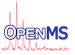OpenMS  2.8.0
HyperScore Struct Reference

An implementation of the X!Tandem HyperScore PSM scoring function. More...

`#include <OpenMS/ANALYSIS/RNPXL/HyperScore.h>`

## Classes

struct  PSMDetail
compute the (ln transformed) X!Tandem HyperScore overload that returns some additional information on the match More...

## Public Types

typedef std::pair< Size, double > IndexScorePair

## Static Public Member Functions

static double compute (double fragment_mass_tolerance, bool fragment_mass_tolerance_unit_ppm, const PeakSpectrum &exp_spectrum, const PeakSpectrum &theo_spectrum)
compute the (ln transformed) X!Tandem HyperScore 1. the dot product of peak intensities between matching peaks in experimental and theoretical spectrum is calculated 2. the HyperScore is calculated from the dot product by multiplying by factorials of matching b- and y-ions More...

static double computeWithDetail (double fragment_mass_tolerance, bool fragment_mass_tolerance_unit_ppm, const PeakSpectrum &exp_spectrum, const PeakSpectrum &theo_spectrum, PSMDetail &d)

## Static Private Member Functions

static double logfactorial_ (const int x, int base=2)
helper to compute the log factorial More...

## Detailed Description

An implementation of the X!Tandem HyperScore PSM scoring function.

## ◆ OpenMS::HyperScore::PSMDetail

 struct OpenMS::HyperScore::PSMDetail

compute the (ln transformed) X!Tandem HyperScore overload that returns some additional information on the match

Collaboration diagram for HyperScore::PSMDetail:
[legend]
Class Members
size_t matched_b_ions
size_t matched_y_ions
double mean_error

## ◆ IndexScorePair

 typedef std::pair IndexScorePair

## ◆ compute()

 static double compute ( double fragment_mass_tolerance, bool fragment_mass_tolerance_unit_ppm, const PeakSpectrum & exp_spectrum, const PeakSpectrum & theo_spectrum )
static

compute the (ln transformed) X!Tandem HyperScore 1. the dot product of peak intensities between matching peaks in experimental and theoretical spectrum is calculated 2. the HyperScore is calculated from the dot product by multiplying by factorials of matching b- and y-ions

Note
Peak intensities of the theoretical spectrum are typically 1 or TIC normalized, but can also be e.g. ion probabilities
Parameters
 fragment_mass_tolerance mass tolerance applied left and right of the theoretical spectrum peak position fragment_mass_tolerance_unit_ppm Unit of the mass tolerance is: Thomson if false, ppm if true exp_spectrum measured spectrum theo_spectrum theoretical spectrum Peaks need to contain an ion annotation as provided by TheoreticalSpectrumGenerator.

## ◆ computeWithDetail()

 static double computeWithDetail ( double fragment_mass_tolerance, bool fragment_mass_tolerance_unit_ppm, const PeakSpectrum & exp_spectrum, const PeakSpectrum & theo_spectrum, PSMDetail & d )
static

## ◆ logfactorial_()

 static double logfactorial_ ( const int x, int base = `2` )
staticprivate

helper to compute the log factorial